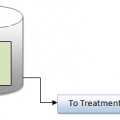## ppm as CaCO3

A method of reporting the concentration or "equivalent" weight of an ion or substance in a given volume of water as "ppm as calcium carbonate". Reporting the concentration of ions as "ppm as calcium carbonate" is popular by ion exchange chemists for the calculation of ionic loading of cation or anion resins. It is also popular in determining whether a water analysis is "balanced" where the sum of the cations equals the sum of the anions when the concentration of the ions are reported as calcium carbonate equivalents. Water chemists use the concept of "equivalency" when balancing cation and anion electroneutrality levels since ions combine in nature based on their valence state and available electrons, not on their "actual" weight. Calcium carbonate was arbitrarily picked because its molecular weight is 100 and its equivalent weight is 50 since its divalent. The formula to convert an ion reported as "mg/l as the ion" to "ppm as calcium carbonate" is to multiply "mg/l as the ion" times the ratio of the "equivalent weight of the ion" by the "equivalent weight of calcium carbonate".

As an example, a water with sodium at 100 ppm as calcium carbonate and chloride at 100 ppm as calcium carbonate are in ionic balance since every sodium ion has a corresponding chloride ion. However, sodium concentration at 100 ppm as calcium carbonate is only 47 mg/l of actual substance (since its equivalent weight is 23.0) and 100 ppm of chloride as calcium carbonate is only 71 mg/l of actual substance (since its equivalent weight is 35.5). The calculated TDS of this solution is 118 mg/l.## Author:T.P.Sivanandan

#### Why Fine Bubbles are Better than Coarse bubble in Aeration Tank#### How to Calculate Lime Dosage Requirement in Sedimentation Process## 5 thoughts on “ppm as CaCO3”

1.Anonymous says:

What is the conversion of:
Calcium 300 mg/L
into
Calcium ppm.

H20wzrd@aol.com

2.Anonymous says:

I think they are the same parts per million = milligrams per litre

3.Anonymous says:

"The formula to convert an ion reported as "mg/l as the ion" to "ppm as calcium carbonate" is to multiply "mg/l as the ion" times the ratio of the "equivalent weight of the ion" by the "equivalent weight of calcium carbonate"."

4.Renuka says: